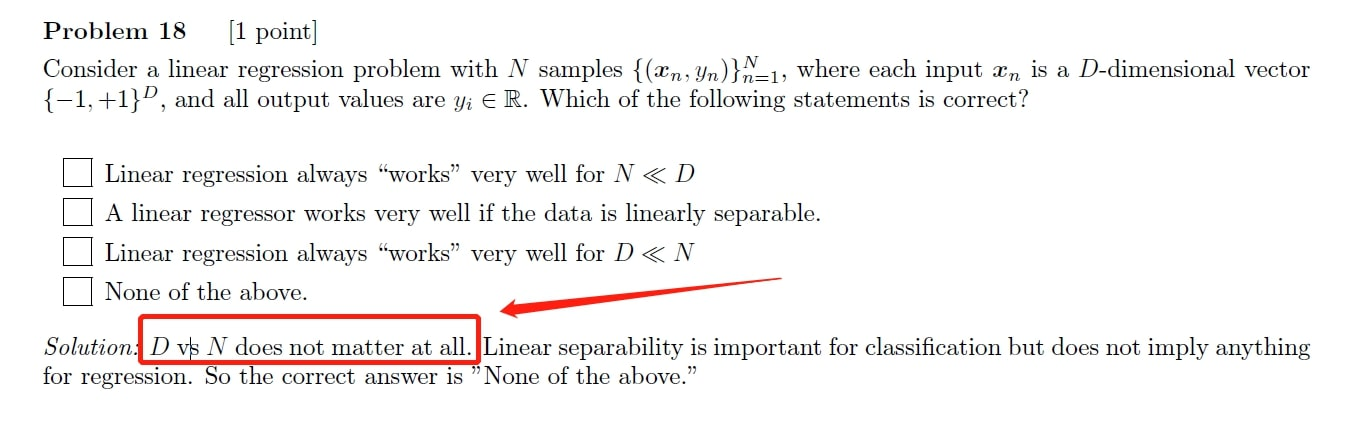### Why "D vs N does not matter at all"? Final 2017 Problem 18

Hi,

We discussed the D>N problem in handout 01c. I don't understand why the answer to Problem 18 says "D vs N does not matter at all".

Thanks!Hi, I had the same problem but I finally draw these conclusions:

In the 1st one you cite, it is written "linear regression", but since y is defined as being {-1, +1} it's a classification problem so indeed if data is linearly separable it will work very well.
In the 2nd one you cite, y is defined as being in R so this time it is a regression, where linearly separable data doesn't make any difference. Then the dimensions doesn't guarantee anything since we don't know if the data has a linear relation which is the assumption needed for linear regression.
What do you think?

Page 1 of 1